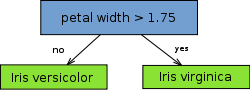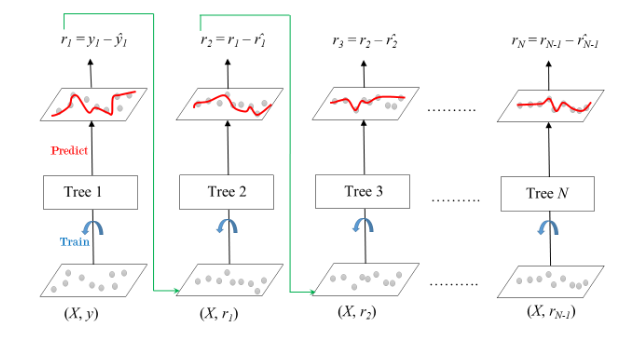×

Search anything:

# Boosting, an Ensemble Method

#### Machine Learning (ML)Get this book -> Problems on Array: For Interviews and Competitive Programming

In this article, we have covered the idea of Boosting in depth along with different types of Boosting algorithms, benefits and challenges of Boosting.

Table of content:

1. Introduction to Ensemble Methods
2. Boosting
3. Boosting Algorithms
4. Improvements to Basic Gradient Boosting
5. Benefits of Boosting
6. Challenges of Boosting
7. Conclusion

Let us get started with boosting.

# Introduction to Ensemble Methods

Maybe almost all of you reading this is familiar with Machine Learning Algorithms such as K Neighbor Classifier or Regressor, Linear Regression, Ridge, etc. But, What if your model is not robust enough as you might say. One of the solutions of that questions is Boosting, an Ensemble Algorithm.

In general, people working in groups are more likely to perform better than individuals working alone. When task are assigned to a group, that particular group could approach the task with different kinds of perspective. The same rule also applies to Machine Learning models. We could group different individual weak learners to achieve a robust, and highly accurate model.

This is what we called Ensemble Methods, a method that combine base estimators to make a single estimator with a higher accuracy than a single estimator.

There are two types of Ensemble Methods, averaging, and boosting. On this particular article, we'll focus on Boosting.

# Boosting

Boosting means combining several weak learners in series. Boosting method mainly focus on training that previous model have gotten wrong. The key property of boosting ensembles is the idea of correcting prediction errors. The models are fit and added to the ensemble sequentially such that the second model attempts to correct the predictions of the first model, the third corrects the second model, and so on.

# Boosting Algorithms

Boosting Algorithms combines each weak learner to create one strong prediction rule. To identify the weak rule, there is a base model. Whenever the model is applied, it creates new prediction rules using the iteration process. After some iteration, it combines all weak rules to create one single prediction rule. There's two major algorithms in Boosting, AdaBoost (Adaptive Boosting), and Gradient Boosting.

AdaBoost stands for Adaptive Boosting, a boosting method used as an Ensemble Method. Adaboost starts by predicting on the original dataset as simple as possible, and then it gives equal weights to each observation. If the prediction made using the first learner is incorrect, it allocates the higher importance to the incorrectly predicted statement and an iterative process. It goes on to add new learners until the limit is reached in the model.One of the most commonly used as a base estimator or weak learner for AdaBoost is Decision Stump.

Decision Stump is a Decision Tree Machine Learning model, but only consists of one internal node, which is immediately connected to the leaves. A decision stump makes a prediction based on the value of just a single input feature.## Implementation in Python

``````from sklearn.ensemble import AdaBoostClassifier #for classifier
from sklearn.ensemble import AdaBoostRegressor #for regressor

clf.fit(X, y)
``````
• It will use Decision Stump as it's base estimator.
• n_estimator parameter used to decide how many weak learners to use.
• Learning Rate parameter controls the contribution of all the vulnerable learners in the final output

Gradient boosting approaches the problem a bit differently. Instead of adjusting weights of data points, Gradient boosting focuses on the difference between the prediction and the ground truth. Gradient Boosting trains many models in a gradual, additive and sequential manner. While the AdaBoost model identifies the shortcomings by using high weight data points, gradient boosting achieves the same effect by using gradients in the loss function.## Implementation in Python

``````from sklearn.ensemble import GradientBoostingClassifier #for classifier
from sklearn.ensemble import GradientBoostingRegressor #for regressor

clf.fit(X, y)
``````
• n_estimator and learning_rate parameters works the same as AdaBoost.

XGBoost is one of the variants of gradient boosting that commonly used. It is a ensemble Machine Learning with decision tree as it's weak learner. XGBoost is designed to enhance the performance and speed of a Machine Learning model. XGBoost uses pre-sorted algorithm and histogram-based algorithm for computing the best split. The histogram-based algorithm splits all the data points for a feature into discrete bins and uses these bins to find the split value of the histogram. Also, in XGBoost, the trees can have a varying number of terminal nodes and left weights of the trees that are calculated with less evidence is shrunk more heavily.

## Improvements to Basic Gradient Boosting

Gradient Boosting is a greedy algorithm and it can overfit a training dataset quickly. There's some improvements to prevent overfitting on Gradient Boosting

1. Tree Constraints

There's some constraints that can be applied on the construction of the decision trees, such as Number of trees, tree depth, Number of nodes, minimum imrpovement to loss.

The contribution of each tree to this sum can be weighted to slow down the learning by the algorithm. This weighting is called a shrinkage or a learning rate. The effect is that learning is slowed down, in turn require more trees to be added to the model, in turn taking longer to train, providing a configuration trade-off between the number of trees and learning rate.

## Benefits of Boosting

1. Boosting models could be tuned with several hyper-parameter options to improve the model. In boosting, we don't have to do data preprocessing before fitting, because in libraries for boosting such as scikit-learn, it has built-in routines to handle missing data.

2. Boosting algorithms combine multiple weak learners iteratively, and improving upon observations. It could reduce high bias that commonly occurred in models such as Logistic Regression and Decision Trees.

3. Boosting Algorithms only select features that have significant effect to the target, so it could help reduce dimensionality as well increase computational efficiency.

## Challenges of Boosting

1. Sequential training is hard to scale up, since each estimator is built based on its predecessors, boosting can be computationally expensive. XGBoost seeks to address scalability issues seen in other types of boosting. Boosting algorithms can be slower compared to bagging, because a large number of parameters can influence the behavior of the model.

# Conclusion

Boosting is an ensemble method that combines weak learners into a strong one. It could outperform shallow Machine Learning models like Logistic Regression. Boosting is also suitable for artificial intelligence projects across range of industries including Healthcare, IT, and Finance.

With this article at OpenGenus, you must have the complete idea of Boosting. Enjoy.